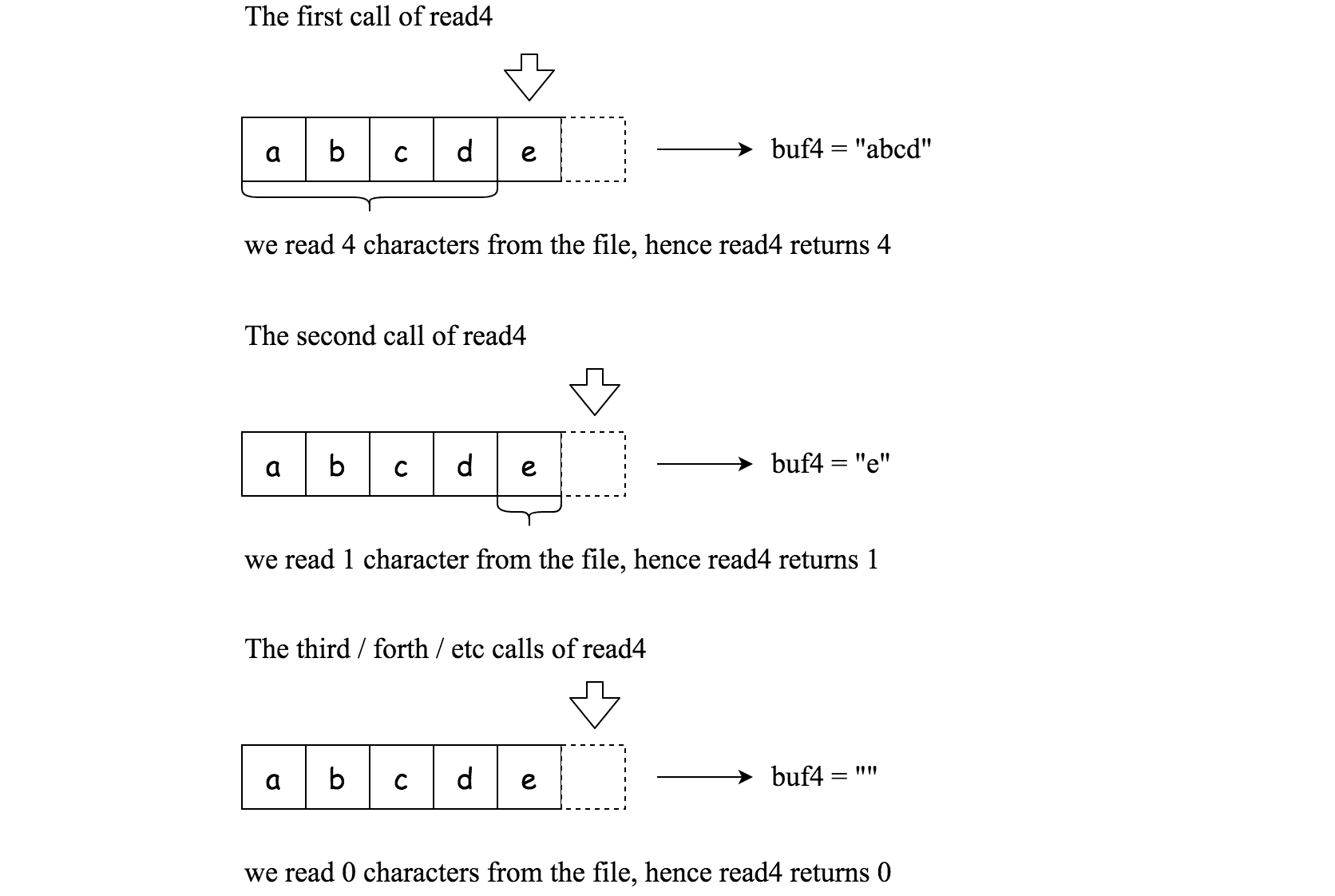# Question

Formatted question description: https://leetcode.ca/all/157.html

Given a file and assume that you can only read the file using a given method read4, implement a method to read n characters.

The API read4 reads four consecutive characters from file, then writes those characters into the buffer array buf4.

The return value is the number of actual characters read.

Note that read4() has its own file pointer, much like FILE *fp in C.

    Parameter:  char[] buf4
Returns:    int

buf4[] is a destination, not a source. The results from read4 will be copied to buf4[].


Below is a high-level example of how read4 works:File file("abcde"); // File is "abcde", initially file pointer (fp) points to 'a'
char[] buf4 = new char; // Create buffer with enough space to store characters
read4(buf4); // read4 returns 4. Now buf4 = "abcd", fp points to 'e'
read4(buf4); // read4 returns 1. Now buf4 = "e", fp points to end of file
read4(buf4); // read4 returns 0. Now buf4 = "", fp points to end of file


# Algorithm

Read every 4, and then judge the results,

• If it is 0, it means that the buf has been read at this time, and the loop is jumped out, and the smaller value of res and n is directly returned.
• Otherwise, keep reading until n characters are read, the loop ends, and finally the smaller value of res and n is returned

# Code

• 
public class Solution extends Reader4 {
/**
* @param buf    Destination buffer
* @param n   　　Maximum number of characters to read
* @return　     The number of characters read
*/

public int read(char[] buf, int n) {
boolean isEof = false;
char[] buf4 = new char;

while (!isEof && charsRead < n) {
if (size < 4) {
isEof = true;
}

if (charsRead + size > n) {
}

/*
arraycopy(Object src,  int  srcPos,
Object dest, int destPos,
int length);
*/
// if last iteration and size==0, then copy length==0, not over-copy
}

}

}

return 1; // stub
}
}
}

############

/**
*/

public class Solution extends Reader4 {
/**
* @param buf Destination buffer
* @param n   Number of characters to read
* @return    The number of actual characters read
*/
public int read(char[] buf, int n) {
char[] buf4 = new char;
int i = 0, v = 5;
while (v >= 4) {
for (int j = 0; j < v; ++j) {
buf[i++] = buf4[j];
if (i >= n) {
return n;
}
}
}
return i;
}
}

• // OJ: https://leetcode.com/problems/read-n-characters-given-read4/
// Time: O(N)
// Space: O(1)
// Forward declaration of the read4 API.
class Solution {
public:
/**
* @param buf Destination buffer
* @param n   Number of characters to read
* @return    The number of actual characters read
*/
int read(char *buf, int n) {
int cnt = 0;
while (cnt < n) {
int x = read4(buf + cnt);
if (!x) break;
cnt += x;
}
cnt = min(cnt, n);
buf[cnt] = '\0';
return cnt;
}
};

• """

@param buf4, a list of characters
@return an integer

# Below is an example of how the read4 API can be called.
file = File("abcdefghijk") # File is "abcdefghijk", initially file pointer (fp) points to 'a'
buf4 = [' '] * 4 # Create buffer with enough space to store characters
read4(buf4) # read4 returns 4. Now buf = ['a','b','c','d'], fp points to 'e'
read4(buf4) # read4 returns 4. Now buf = ['e','f','g','h'], fp points to 'i'
read4(buf4) # read4 returns 3. Now buf = ['i','j','k',...], fp points to end of file
"""

class Solution:
"""
:type buf: Destination buffer (List[str])
:type n: Number of characters to read (int)
:rtype: The number of actual characters read (int)
"""
i = 0
buf4 =  * 4
v = 5
while v >= 4:
for j in range(v):
buf[i] = buf4[j]
i += 1
if i >= n:
return n
return i

############

# @param buf, a list of characters
# @return an integer

class Solution(object):
"""
:type buf: Destination buffer (List[str])
:type n: Maximum number of characters to read (int)
:rtype: The number of characters read (int)
"""
cnt = 0
tmp = [""] * 4
while cnt < n:
if r == 0:
break
for i in range(min(r, n - cnt)):
buf[cnt] = tmp[i]
cnt += 1
return cnt


• /**
*
*     read4 := func(buf4 []byte) int
*
* // Below is an example of how the read4 API can be called.
* file := File("abcdefghijk") // File is "abcdefghijk", initially file pointer (fp) points to 'a'
* buf4 := make([]byte, 4) // Create buffer with enough space to store characters
* read4(buf4) // read4 returns 4. Now buf = ['a','b','c','d'], fp points to 'e'
* read4(buf4) // read4 returns 4. Now buf = ['e','f','g','h'], fp points to 'i'
* read4(buf4) // read4 returns 3. Now buf = ['i','j','k',...], fp points to end of file
*/

var solution = func(read4 func([]byte) int) func([]byte, int) int {
return func(buf []byte, n int) int {
buf4 := make([]byte, 4)
i, v := 0, 5
for v >= 4 {
for j := 0; j < v; j++ {
buf[i] = buf4[j]
i++
if i >= n {
return n
}
}
}
return i
}
}